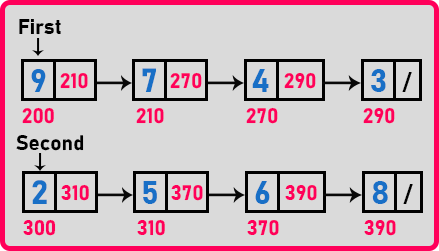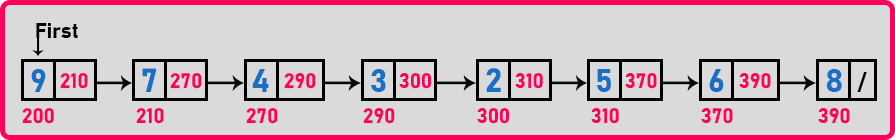# How to Concatenate two Linked Lists

## How to Concatenate two Linked Lists in C Language with Examples:

In this article, I am going to discuss How to Concatenate two Linked Lists in C Language with Examples. Please read our previous article, where we discussed the Recursive Procedure for Reversing a Linked List in C Language with Examples.

##### How to Concatenate two Linked Lists?

Here, we will look at the concatenation of two linked lists.Here we have two linked lists. Concatenation means joining two linked lists or appending one linked list to another linked list. Here we want to append the second linked list to the first linked list. It can be done in another way also i.e. we can append the first linked list to the second linked list. So, it is just like doing first plus second and we want to generate a combined linked list. Let us look at the procedure.

##### Procedure for Concatenate two Linked Lists:

We have to reach the end of the first linked list and instead of null it should point to the first node of the second linked list, so it becomes a complete single linked list.Here, we will take a pointer ‘p’ and traverse the whole linked list until the p pointer reaches the last node.Now how do we know that node is the last node? Because that node’s next is null. So, we will stop at the node whose next pointer is null. And once we reach that node, we will join these two linked lists. Now let us write pseudo code for the concatenation of two linked lists.

##### Pseudo Code for Concatenate two Linked Lists:

p = first;
while(p->next != NULL){
p = p->next;
}
p->next = second;
second = NULL;

Now let us write the complete program.

##### Program for Concatenate two Linked Lists in C Language:
```#include <stdio.h>
#include <stdlib.h>
struct Node
{
int data;
struct Node *next;
} *temp = NULL, *first = NULL, *second = NULL;

struct Node* Create(int A[], int n)
{
int i;
struct Node *t, *last;
temp = (struct Node *) malloc(sizeof(struct Node));
temp->data = A;
temp->next = NULL;
last = temp;

for (i = 1; i < n; i++)
{
t = (struct Node *) malloc(sizeof(struct Node));
t->data = A[i];
t->next = NULL;
last->next = t;
last = t;
}
return temp;
}

void Display(struct Node *p)
{
while (p != NULL)
{
printf ("%d ", p->data);
p = p->next;
}
}

void Concat(struct Node *first, struct Node *second)
{
struct Node *p = first;
while (p->next != NULL)
{
p = p->next;
}
p->next = second;
second = NULL;
}

int main()
{
int A[] = { 9, 7, 4, 3 };
int B[] = { 2, 5, 6, 8 };
first = Create(A, 4);
second = Create(B, 4);

Display (first);

Display (second);

Concat (first, second);